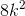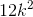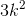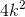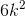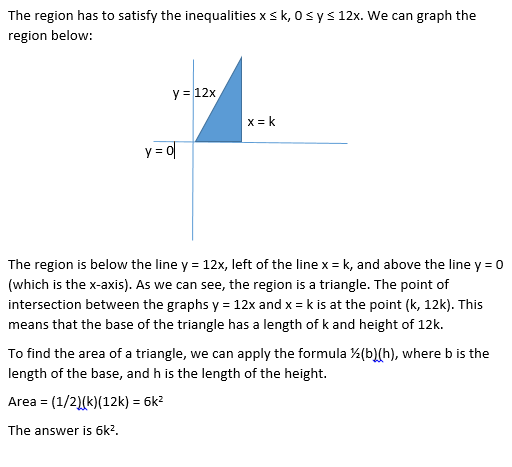# PSAT Math : How to graph a two-step inequality

## Example Questions

### Example Question #1 : Graphing

Let D be the region on the (x,y) coordinate plane that contains the solutions to the following inequalities: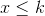, whereis a positive constant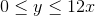Which of the following expressions, in terms of, is equivalent to the area of D?